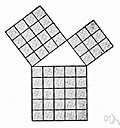Also found in: Thesaurus, Wikipedia.
ThesaurusAntonymsRelated WordsSynonymsLegend:
 Noun 1matrix addition - the addition of matrices    matrix operation - a mathematical operation involving matrices
Based on WordNet 3.0, Farlex clipart collection. © 2003-2012 Princeton University, Farlex Inc.
Mentioned in ?
References in periodicals archive ?
According to GSW, due to the presence of noise, it needs to introduce a number of operations to achieve the FHE scheme, such as BitDecomp(), [BitDecomp.sup.-1](), Powersof 2(), matrix addition, matrix multiplication and so on.
In the GSW-FHE scheme, homomorphic addition and multiplication are just matrix addition and multiplication.
It contains matrix multiplication and matrix addition, which are preferable in hardware implementation.
The Matrix sum is used to accumulate the two signals using matrix addition process.
We solve the matrix operation identification problem for matrix multiplication of different kinds as well as matrix addition and subtraction.
In the case of matrix addition, we have A + B [member of] H(T, 2k); the rank of an exact matrix product is less obvious.

Site: Follow: Share:
Open / Close